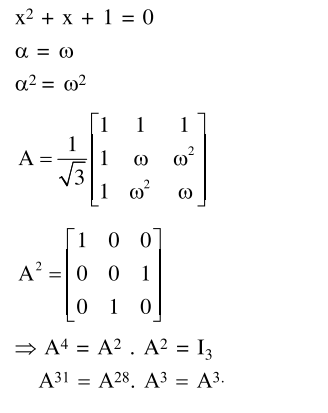# Let α be a root of the equation

Question:

Let $\alpha$ be a root of the equation $x^{2}+x+1=0$

and the matrix $A=\frac{1}{\sqrt{3}}\left[\begin{array}{ccc}1 & 1 & 1 \\ 1 & \alpha & \alpha^{2} \\ 1 & \alpha^{2} & \alpha^{4}\end{array}\right]$, then the matrix $A^{31}$ is equal to:

1. $\mathrm{A}^{3}$

2. $\mathrm{A}$

3. $\mathrm{A}^{2}$

4. $\mathrm{I}_{3}$

Correct Option: 1

Solution: